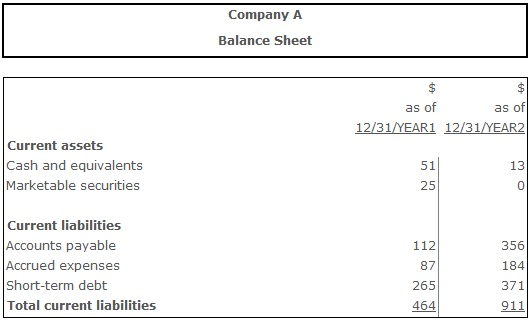# Cash Ratio

Cash Ratio – a ratio estimating the share of the company's current liabilities that can be paid off immediately. It can be calculated by dividing the firm's most liquid assets by its current (short-term) liabilities. The most liquid part of assets includes cash and its equivalents (marketable securities, etc.).

Normative is the cash ratio value that lies in a range between 0,1 and 0,2. Lower values indicate available problems with covering company's current liabilities immediately, since company doesn't possess enough cash and equivalents to do that. Higher than normative cash ratio can witness company's inefficient asset structure. Cash and equivalents usually are the least productive assets of a company, and just their availability on firm's account does not generate any profit. Even converting them to the form of short-term investments would generate some additional minor income. That's why too high value of the cash ratio indicates that a big part of assets is not being productive.

Talking about lower than normative cash ratio analysts should pay close attention to company's financial planning process. If it is developed well enough, company's management would be able to measure the exact amount of money available on current accounts at any time. In this case keeping a lot of cash and equivalents on accounts wouldn't be reasonable, and lower than normative cash ratio would not indicate the risk of losing the liquidity.

## Resolving the problems with the cash ratio exceeding the normative range:

If the cash ratio value is lower than normative, the firm can involve loans or realize a part of exceeding assets in order to increase the amount of the most liquid assets. If the cash ratio value is higher than normative, the company can invest a part of the exceeding funds in operations, financial investments, etc.

## Formula(s):

Cash Ratio = (Cash Equivalents + Marketable Securities) ÷ Current Liabilities

## Example:Cash Ratio (Year 1) = (51 + 25) ÷ 464 = 0,16 = 16%

Cash Ratio (Year 2) = (13 + 0) ÷ 911 = 0,01= 1%

Cash ratio was 0,16 (16%) in year 1. This means that the company was able to pay immediately 16% of its current obligations. In year 2 cash ratio has decreased to the level of 0,01 (1%), meaning the ability of a firm to meet its current obligations has become lower. In year 2 it most likely would face problems meeting its current obligations immediately.

## Conclusion:

Cash ratio shows the relationship between the most liquid assets of a company and its current liabilities and measures the ability of a company to meet its obligation immediately. Lack of the necessary amount of cash and equivalents would cause the necessity of involving additional credit resources, selling some of it's less liquid assets for lower price, activation of the accounts receivable collecting etc.